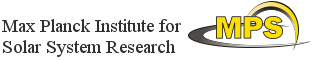## Exploitation of Space Data for Innovative Helio- and Asteroseismology

### Helioseismology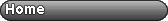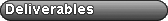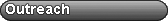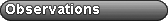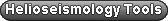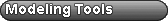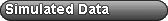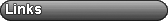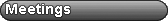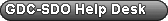# Calculating Ingression & Egression for Holography

An illustration of how the magnetic fields rotate
with the Sun to the far side, where the inset shows the
holographic image of the backside.    SOHO-HotShots

Helioseismic holography (in very simple terms) takes the observed oscillations on the Sun's surface and calculates the 'seismic image' at a given point at some depth in the Sun. When this is calculated forward in time, it is called the "egression" and when the calculation is reversed in time it is called the "ingression". This is explained in more detail in the paper by Lindsey, C. and Braun, D.C., 2000, Basic Principles of Solar Acoustic Holography, Sol. Phys., 192, 261.

The programs, "regress_plane_calc.c" and "regress_plane_calc_eikonal.c" were written by C. Lindsey to compute egressions and ingressions for helioseismic holography (Lindsey, C. and Braun, D.C., 2000, Basic Principles of Solar Acoustic Holography, Sol. Phys., 192, 261). The former uses the full hydromechanical calculation and the latter uses the eikonal equation.

We encourage people interested in getting into holography to use "regress_plane_eikonal" instead of "regress_plane". The two are very similar for pupils more than a few pixels in radius, but for relatively small pupils, "regress_plane_eikonal" is more stable, for various technical reasons.

Both codes require similar input. The keywords expected in the header of the input data are something like this.

INPUT PARAMETERS: Requires the input data (a four-dimensional FITS datacube where the first two dimensions are spatial, the third is frequency and the fourth is the real and imaginary components), the Green's functions (space-frequency), which pupil to use, the user to specify whether to calculate the egression (-e) and/or ingression (-i) and the name of the output file. A complete list and explanations can be garnered by typing "regress_plane_calc_eikonal" in the command line after compiling.

*The program requires the pupil to be a 2-dimensional FITS file with the same spatial scaling as the input datacube. Download an example pupil (right click).

OUTPUT: The program outputs a 4-dimensional FITS cube of the regression, based on the parameter choices.

webmaster